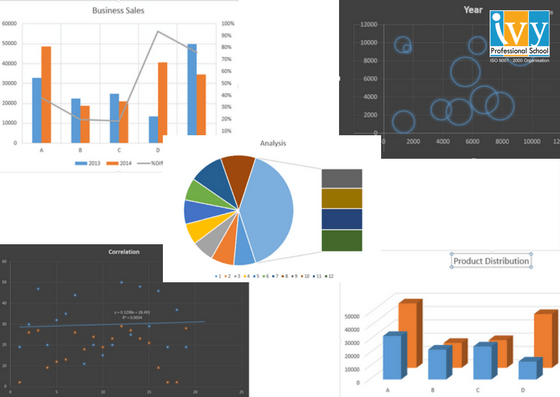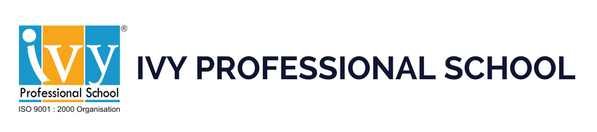# Commonly used Business Analytics Techniques

Statistics is the backbone of Analytics. Over the course of time considering the huge amount and various types of data simple statistical measures have proved inconsistent. Thus various advanced business analytics tools have been developed using statistical methods such as regression, time series, clustering, forecasting which are not only able to handle large data but are also efficient in predicting better business insights.Used to determine the strength of relationship which a dependent variable has with a series of other independent variables, regression technique is used for predictive analysis in almost every business sector. Linear and logistic regressions are the two most commonly used regression techniques that anyone generally learns.

Forecasting is used for determining the future trend for a business, based on past data. Clustering is another important technique considering the huge amount of data being used to analyse a business.

Given the high demand for the knowledge of these statistical methods and their proper implementation using commonly used tools brings the necessity to enrol into the best data science courses in India.

Types of Business Analytics Techniques an analyst must be aware of: –

1. Linear Regression: – As the name suggests, Linear regression is the linear relationship shared between a continuous dependent variable and one or more independent variables. When the number of independent variable is one, it is called simple linear regression and if the number of independent variable is more than one it is called the multiple regression model. Designed more than 250 years ago, this method is one of the most commonly used regression methods. Linear regression is highly sensitive to outliers, assumes that the data is independent and is limited to linear relationships.
2. Logistic regression: – A linear regression model requires the data to be linear in nature. But when the data is categorical in nature, linear regression fails. For the data with the dependent variable binary in nature, logistic regression is used. One of the biggest advantage of logistic regression is that you can include more than one explanatory/dependent variable. Although it is the second most commonly used regression methods, logistic regression cannot predict continuous outcomes and can have multicollinearity.
3. Clustering: – Often the data being used for analysis is scattered and ungrouped. Clustering is a business analytics technique that is used to group the scattered data based on similar properties from others. This is one of the best advantages of clustering as when the amount of data is very large, approaching individual data is less efficient. Though the main advantage of a clustered solution is automatic recovery from failure, in case of data corruption, clustering isn’t efficient in data recovery. Moreover clustering is a complex process.
4. Time-Series: – Businesses often require to statistically predict and estimate some future event or its occurrence economically, technically or demand wise. Time-series forecasting is a statistical method that helps businesses make accurate predictions based on past and present data and most commonly by analysis of trends. As the name suggests a time series is a sequence of observations taken sequentially in time. Forecasting is also sought to be the best way to get rid of the variation over time effect. Although it is one of the best techniques even being employed in machine learning, it is often neglected because of the complexities the time component brings with it.

These are just few but most commonly used techniques. Each business analytical tool has its own advantage and disadvantage. So a proper practice and knowledge of each of the business analytics techniques is important. It’s important for an analyst to know which kind of data needs which kind of analysis.